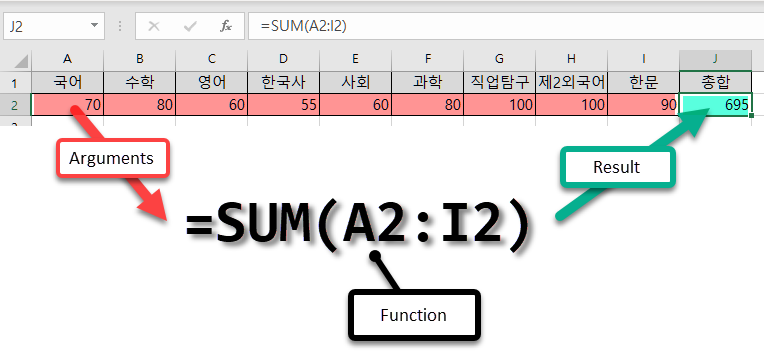microsoft-office

# Excel: How to use functions## 1_Function basics

Functions are convenient functions of Excel. It can be said to be the basics of Excel with everything from basic functions such as addition, subtraction, multiplication, and division to complex and powerful functions.

The function consists of the function_name(argument), and the result is displayed in the cell where the function formula is entered. The usage is the same for any other function.

=SUM(A2:I2) means as below.

## 2_arithmetic operator

Arithmetic operators that can be used in Excel are as follows.

It is also possible to use a combination of the arithmetic operators described above.

As you learned in math class, you start with the parentheses ().

## 3_comparison operator

Comparison operators that can be used in Excel are as follows.

In addition to numbers, you can also enter regular characters.

(You can see that large and small are determined according to the order of the letters)